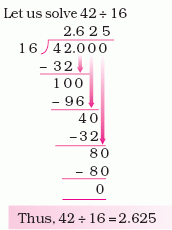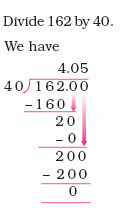Name: ___________________Date:___________________

 Email us to get an instant 20% discount on highly effective K-12 Math & English kwizNET Programs!

### Grade 5 - Mathematics4.7 Division of a Whole Number by Whole Number (Other Than Zero)

Method:
• When the divisor is greater than the dividend we mark a decimal point in both the quotient and dividend
• Continue the division.Directions: Using the above method, find the quotient in each of the following and show your work. Also write at least 5 examples of your own.
 Q 1: Divide 20 by 50.Answer: Q 2: Divide 8 by 100.Answer: Q 3: Divide 45 by 125.Answer: Q 4: Divide 72 by 576.Answer: Q 5: Find the quotient for: 144 ÷ 15Answer: Q 6: Find the quotient for: 67 ÷ 25Answer: Question 7: This question is available to subscribers only! Question 8: This question is available to subscribers only!